# Creating an SQLite Custom CRUD Class in PHP Tutorial

In this tutorial, you will learn how to create a Custom CRUD Class of SQLite Queries using PHP Language. This tutorial aims to provide IT/CS students and new programmers with a reference for enhancing their knowledge and programming capabilities using PHP and SQLite3 Database. Here, snippets for achieving the objectives of this tutorial are provided. Also, a working sample source code zip file that demonstrates the usage of the Custom CRUD Class is provided and free to download.

## What is the purpose of Custom SQLite CRUD Class in PHP?

The Custom SQLite Class in PHP is a simple PHP object that developers can use for handling database queries and execution in a PHP Project. Since the object is for CRUD (Create, Read, Update, and delete) operations of an application or program, the object contains the methods for simplifying the queries for the said operations. It can help developers to write codes or develop their projects easier and faster.

## How to Create an SQLite Custom CRUD Class in PHP?

Well, PHP version 5 or later version comes with SQLite3 class which is an object that interfaces SQLite version 3 Database. You can enable this class or object by enabling the sqlite3 extension on your php.ini file. See the following image for enabling or uncommenting the said extension: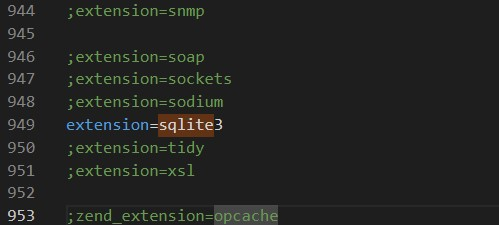Next, before we create the custom CRUD class, we must create first the database connection object for the application and the SQLite3. The following snippet is an example of creating the SQLite3 Database Connection in PHP. The database connection object provided below contains also the method that creates the database table.

`    <?php    class Database extends SQLite3 {        public function __construct(\$db_file_path=""){           if(empty(\$db_file_path)){                throw new ErrorException("SQLite DB file is not set.");                exit;            }            \$this->open(\$db_file_path);            if(\$this->lastErrorCode() > 0){                throw new ErrorException("Database Connection failed.");                exit;            }            \$this->create_post_table();        }         public function create_post_table(){            /**             * Creating a sample post table             */            \$post_tbl = "CREATE TABLE IF NOT EXISTS `posts`                         (                            `id` INTEGER NOT NULL PRIMARY KEY AUTOINCREMENT,                            `title` text NOT NULL,                            `meta_description` text NOT NULL,                            `content` text NULL DEFAULT NULL,                            `created_at` datetime DEFAULT CURRENT_TIMESTAMP,                            `updated_at` datetime NULL DEFAULT NULL                        )                        ";            \$this->exec(\$post_tbl);            if(\$this->lastErrorCode()){                throw new ErrorException(\$this->lastErrorMsg(), \$this->lastErrorCode());            }            /**             * POST update datetime trigger             */            \$post_dt_trigger = "CREATE TRIGGER IF NOT EXISTS [UpdateAT]                AFTER                UPDATE                ON `posts`                FOR EACH ROW                BEGIN                    UPDATE `posts` set `updated_at` = CURRENT_TIMESTAMP where `id`=OLD.`id`;                END            ";            \$this->exec(\$post_dt_trigger);            if(\$this->lastErrorCode()){                throw new ErrorException(\$this->lastErrorMsg(), \$this->lastErrorCode());            }        }         public function __destruct(){            /**             * destruct the sample class object             */            \$this->close();        }    }    if(!is_dir("./database"))    mkdir("./database");    // \$database = new Database(); `

Next, we'll now create the custom CRUD class or object. The object must derive the SQLite3 class or the Custom SQLite3 Database class so we can access the properties of the SQLite for executing the database queries. Check out the snippet below.

`<?php class DBCRUD extends DATABASE{    public function insert(\$tbl="", \$data = []){        if(empty(\$tbl)){            throw new ErrorException("Insertion Table Name must be specified.");        }        if(empty(\$data)){            throw new ErrorException("Insertion required column and data must be specified.");        }         \$insert_columns = [];        \$insert_values = [];        foreach(\$data as \$k => \$v){            if(!is_numeric(\$v) && !is_array(\$data[\$k]) && !empty(\$v)){                \$v = \$this->escapeString(addslashes(\$v));            }            \$insert_columns[] = \$k;            \$insert_values[] = \$v;        }         \$tbl_columns = implode("`, `", \$insert_columns);        \$tbl_values = implode("', '", \$insert_values);        \$insert_sql = "INSERT INTO `{\$tbl}`                     (`{\$tbl_columns}`)                    VALUES                    ('{\$tbl_values}')            ";        try{            \$this->exec(\$insert_sql);            if(!\$this->lastErrorCode()){                \$resp['status'] = "success";                \$last_id = \$this->lastInsertRowid();                \$resp['id'] = \$last_id;            }else{                throw new ErrorException(\$this->lastErrorMsg(), \$this->lastErrorCode());            }        }catch(Exception \$e){            \$resp['status'] = "failed";            \$resp['error'] = \$e->getMessage();         }        return \$resp;    }    public function update(\$tbl="", \$data = [],\$where=[]){        if(empty(\$tbl)){            throw new ErrorException("Update Table Name must be specified.");        }        if(empty(\$data)){            throw new ErrorException("Update required column and data must be specified.");        }         if(empty(\$where)){            throw new ErrorException("Update Where Array must be specified.");        }        \$update_data= "";        foreach(\$data as \$k => \$v){            if(!is_numeric(\$v) && !is_array(\$data[\$k]) && !empty(\$v)){                \$v = \$this->escapeString(addslashes(\$v));            }            if(!empty(\$update_data)) \$update_data .= ", ";            \$update_data .= "`{\$k}`='{\$v}'";        }        \$where_data= "";        foreach(\$where as \$k => \$v){            if(!is_numeric(\$v) && !is_array(\$where[\$k]) && !empty(\$v)){                \$v = \$this->escapeString(addslashes(\$v));            }            \$type = "and";            \$compare = "=";            \$value = \$v;            if(is_array(\$v)){                if(isset(\$v['value']))                \$value = \$v['value'];                if(isset(\$v['type']))                \$type = \$v['type'];                if(isset(\$v['compare']))                \$compare = \$v['compare'];            }            if(!empty(\$where_data)) \$where_data .= " {\$type} ";            \$where_data .= "`{\$k}` {\$compare} ".(!empty(\$value) ? "'{\$value}'" : "");        }         \$update_sql = "UPDATE `{\$tbl}` set {\$update_data} WHERE {\$where_data}";        try{            \$this->exec(\$update_sql);            if(!\$this->lastErrorCode()){                \$resp['status'] = "success";            }else{                throw new ErrorException(\$this->lastErrorMsg(), \$this->lastErrorCode());            }        }catch(Exception \$e){            \$resp['status'] = "failed";            \$resp['error'] = \$e->getMessage();         }        return \$resp;    }    public function delete(\$tbl="", \$where=[]){        if(empty(\$tbl)){            throw new ErrorException("Table Name for deleting must be specified.");        }        if(empty(\$where)){            throw new ErrorException("Where Array for deleting must be specified.");        }        \$where_data= "";        foreach(\$where as \$k => \$v){            if(!is_numeric(\$v) && !is_array(\$where[\$k]) && !empty(\$v)){                \$v = \$this->escapeString(addslashes(\$v));            }            \$type = "and";            \$compare = "=";            \$value = \$v;            if(is_array(\$v)){                if(isset(\$v['value']))                \$value = \$v['value'];                if(isset(\$v['type']))                \$type = \$v['type'];                if(isset(\$v['compare']))                \$compare = \$v['compare'];            }            if(!empty(\$where_data)) \$where_data .= " {\$type} ";            \$where_data .= "`{\$k}` {\$compare} ".(!empty(\$value) ? "'{\$value}'" : "");        }        \$delete_sql = "DELETE FROM `{\$tbl}` where {\$where_data}";        try{            \$this->exec(\$delete_sql);            if(!\$this->lastErrorCode()){                \$resp['status'] = "success";            }else{                throw new ErrorException(\$this->lastErrorMsg(), \$this->lastErrorCode());            }        }catch(Exception \$e){            \$resp['status'] = "failed";            \$resp['error'] = \$e->getMessage();         }        return \$resp;    }    public function getSingle(\$tbl, \$where=[], \$select = []){        if(empty(\$tbl)){            throw new ErrorException("Table Name for fetching data must be specified.");        }        if(empty(\$where)){            throw new ErrorException("Where Array for fetching data must be specified.");        }        \$where_data= "";        foreach(\$where as \$k => \$v){            if(!is_numeric(\$v) && !is_array(\$where[\$k]) && !empty(\$v)){                \$v = \$this->escapeString(addslashes(\$v));            }            \$type = "and";            \$compare = "=";            \$value = \$v;            if(is_array(\$v)){                if(isset(\$v['value']))                \$value = \$v['value'];                if(isset(\$v['type']))                \$type = \$v['type'];                if(isset(\$v['compare']))                \$compare = \$v['compare'];            }            if(!empty(\$where_data)) \$where_data .= " {\$type} ";            \$where_data .= "`{\$k}` {\$compare} ".(!empty(\$value) ? "'{\$value}'" : "");        }        \$select_column = "";        if(!empty(\$select)){            foreach(\$select as \$k => \$v){                if(!is_numeric(\$v) && !is_array(\$select[\$k]) && !empty(\$v)){                    \$v = \$this->escapeString(addslashes(\$v));                }                if(is_array(\$v) && is_numeric(\$v) && empty(\$v))                continue;                if(!empty(\$select_column)) \$select_column .= ", ";                \$select_column .= "`{\$v}`";            }        }else{            \$select_column = "*";        }        \$selectSingle_sql = "SELECT {\$select_column} FROM `{\$tbl}` WHERE {\$where_data} ";        // return \$selectSingle_sql;        \$query = \$this->query(\$selectSingle_sql);        \$data = \$query->fetchArray(SQLITE3_ASSOC);        return !empty(\$data) ? \$data : [];    }    public function getResults(\$tbl, \$select = [], \$where=[], \$additional_qry = ""){        if(empty(\$tbl)){            throw new ErrorException("Table Name for fetching data must be specified.");        }        \$where_data= "";        foreach(\$where as \$k => \$v){            if(!is_numeric(\$v) && !is_array(\$where[\$k]) && !empty(\$v)){                \$v = \$this->escapeString(addslashes(\$v));            }            \$type = "and";            \$compare = "=";            \$value = \$v;            if(is_array(\$v)){                if(isset(\$v['value']))                \$value = \$v['value'];                if(isset(\$v['type']))                \$type = \$v['type'];                if(isset(\$v['compare']))                \$compare = \$v['compare'];            }            if(!empty(\$where_data)) \$where_data .= " {\$type} ";            \$where_data .= "`{\$k}` {\$compare} ".(!empty(\$value) ? "'{\$value}'" : "");        }        \$select_column = "";        if(!empty(\$select)){            foreach(\$select as \$k => \$v){                if(!is_numeric(\$v) && !is_array(\$select[\$k]) && !empty(\$v)){                    \$v = \$this->escapeString(addslashes(\$v));                }                if(is_array(\$v) && is_numeric(\$v) && empty(\$v))                continue;                if(!empty(\$select_column)) \$select_column .= ", ";                \$select_column .= "`{\$v}`";            }        }else{            \$select_column = "*";        }        \$select_sql = "SELECT {\$select_column} FROM `{\$tbl}` ".(!empty(\$where_data) ? " WHERE {\$where_data} " : "");        if(!empty(\$additional_qry))        \$select_sql .= " {\$additional_qry}";        // return \$select_sql;        \$query = \$this->query(\$select_sql);        \$data = [];        while(\$row = \$query->fetchArray(SQLITE3_ASSOC)){            \$data[] = \$row;        }        return !empty(\$data) ? \$data : [];    }}\$dbcrud = new DBCRUD("./database/test.db");?>`

In the snippet above, the DBCRUD class represents the custom CRUD class that derives from the Custom SQLite Database Class called Database class. The class contains multiple methods that can be used for inserting, updating, reading/fetching, and deleting data from the database. Using the object methods, executing queries will be easier.

### Object Parameters Usage

#### Table Parameter [\$tbl]

This parameter is required for all methods listed on the object. It requires you to enter the database table name you want to manage or where you will execute the database query.

#### Data Parameter [\$data]

This parameter is required in the insert and update method of the object. The parameter contains an array of data to insert or update on the database table. The array key must match the column name on the table and the value as the value.

#### Where Parameter [\$where]

This parameter is required in the update, getSingle, and delete methods but optional in getResults method. It is an array of data for matching the data to manage on the table.

Array Format

`<?php \$where = ["id" => 1];?>`

Or

`<?php\$where = array(     "id" => array(        "value"=>1,        "compare" => "="    ),     "title" => array(        "value"=>"%sample%",        "compare" => "LIKE",        "type" => "OR"    ) );?>`

#### Select Parameter [\$select]

This parameter is an array of table column names to select.

This parameter is a string you wish to add in getResults method query statement such as the "Order By" clause.

### Example

I have provided a working application source code zip file on this site. The application is a simple application with CRUD features. It demonstrates the usage of the Custom SQLite3 CRUD Class that I provided above. The download button is located below this article's content.

#### Application Snapshots

Here are the snapshots of some interfaces of the sample application I have provided.

List Page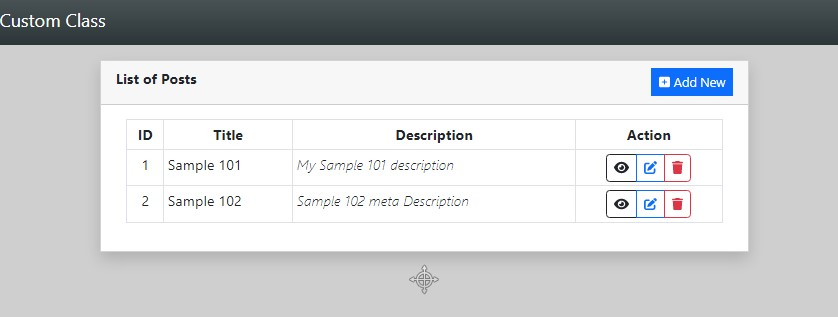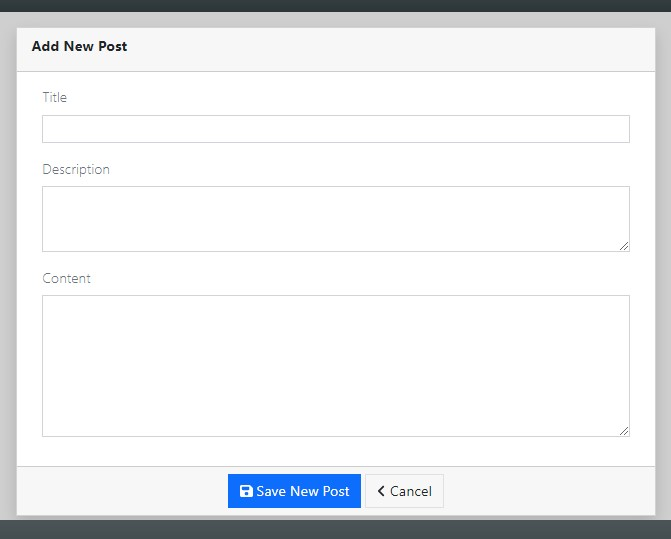Edit Form Page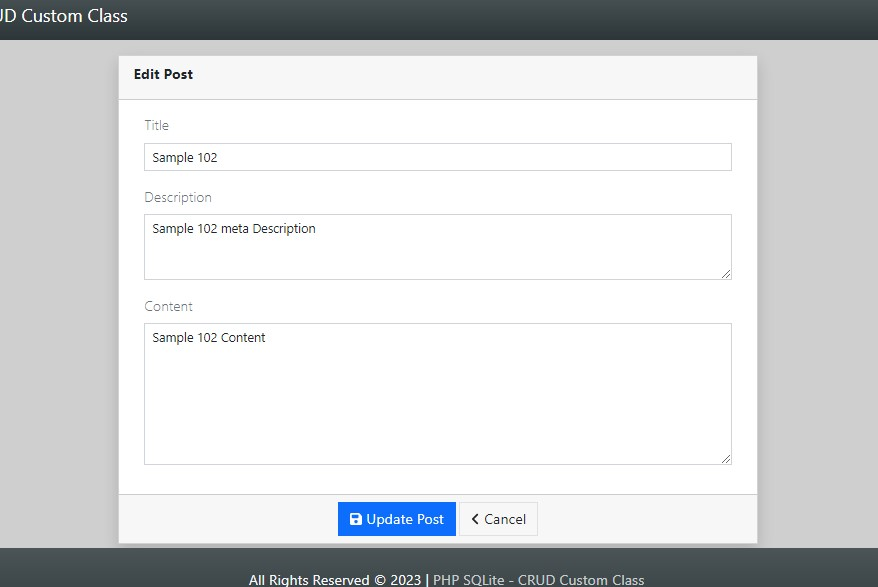View Page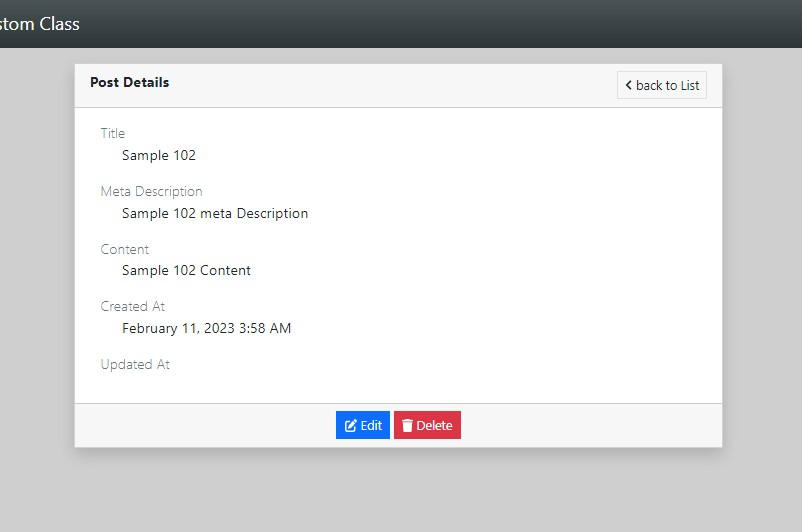### DEMO VIDEO

That's it! I hope this Creation of an SQLite3 Custom CRUD Class in PHP Tutorial will help you with what you are looking for and will be useful for your current and future PHP Projects.

Explore more on this website for more Tutorials and Free Source Codes.

### Happy Coding =)

Submitted byoleteacheron Thu, 02/16/2023 - 08:31

### Thank you, thank you!

Thank you, thank you!

We enjoy your SQLite tutorials and use them in the classroom often.

Please keep them flowing to us:)

Submitted byvaz rwanda (not verified)on Thu, 03/02/2023 - 17:26

very nice app